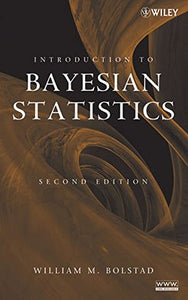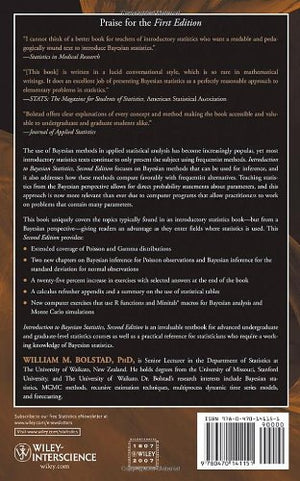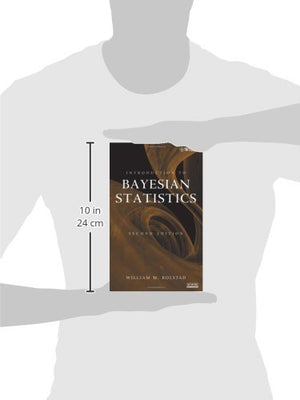# Introduction To Bayesian Statistics, 2Nd Edition

• Publish Date: 2007-08-15
• Binding: Hardcover
• Author: William M. Bolstad
Regular price \$193.63 Sale price \$45.95

Attention: For textbook, access codes and supplements are not guaranteed with used items.

Praise for the First Edition

I cannot think of a better book for teachers of introductory statistics who want a readable and pedagogically sound text to introduce Bayesian statistics.
Statistics in Medical Research

[This book] is written in a lucid conversational style, which is so rare in mathematical writings. It does an excellent job of presenting Bayesian statistics as a perfectly reasonable approach to elementary problems in statistics.
STATS: The Magazine for Students of Statistics, American Statistical Association

Bolstad offers clear explanations of every concept and method making the book accessible and valuable to undergraduate and graduate students alike.
Journal of Applied Statistics

The use of Bayesian methods in applied statistical analysis has become increasingly popular, yet most introductory statistics texts continue to only present the subject using frequentist methods. Introduction to Bayesian Statistics, Second Edition focuses on Bayesian methods that can be used for inference, and it also addresses how these methods compare favorably with frequentist alternatives. Teaching statistics from the Bayesian perspective allows for direct probability statements about parameters, and this approach is now more relevant than ever due to computer programs that allow practitioners to work on problems that contain many parameters.

This book uniquely covers the topics typically found in an introductory statistics bookbut from a Bayesian perspectivegiving readers an advantage as they enter fields where statistics is used. This Second Edition provides:

• Extended coverage of Poisson and Gamma distributions

• Two new chapters on Bayesian inference for Poisson observations and Bayesian inference for the standard deviation for normal observations

• A twenty-five percent increase in exercises with selected answers at the end of the book

• A calculus refresher appendix and a summary on the use of statistical tables

• New computer exercises that use R functions and Minitab macros for Bayesian analysis and Monte Carlo simulations

Introduction to Bayesian Statistics, Second Edition is an invaluable textbook for advanced undergraduate and graduate-level statistics courses as well as a practical reference for statisticians who require a working knowledge of Bayesian statistics.